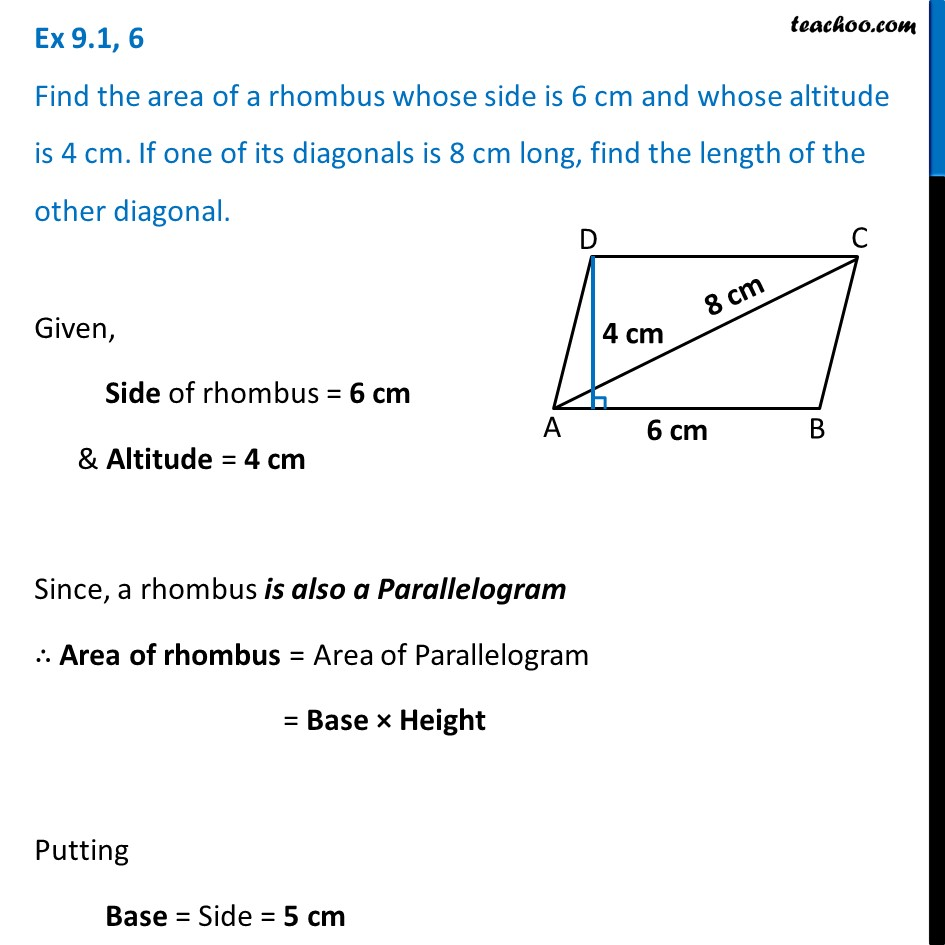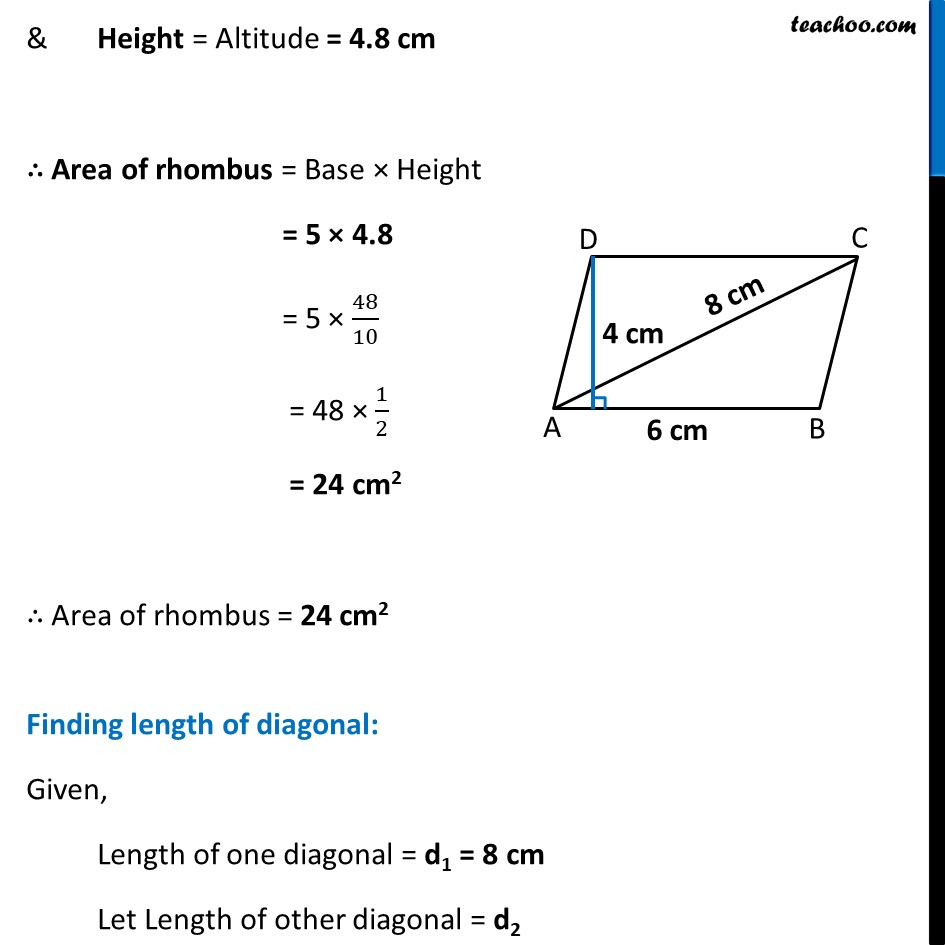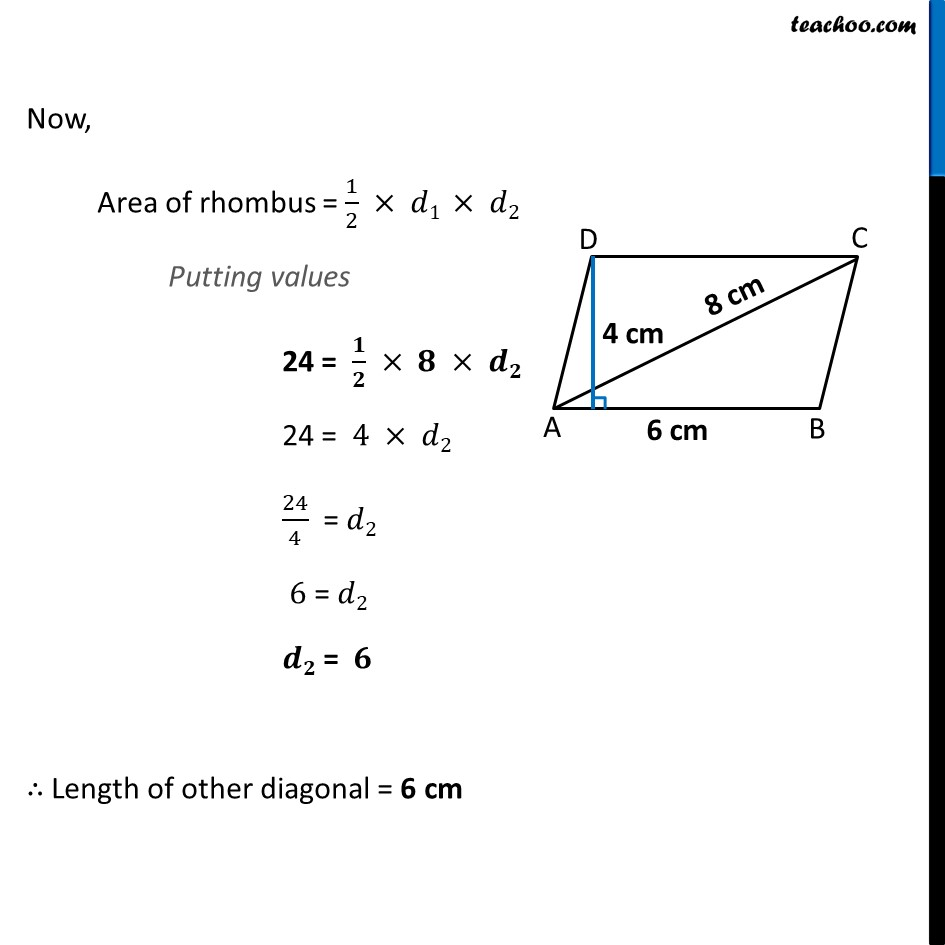Ex 9.1

Chapter 9 Class 8 Mensuration
Serial order wiseLearn in your speed, with individual attention - Teachoo Maths 1-on-1 Class

### Transcript

Ex 9.1, 6 Find the area of a rhombus whose side is 6 cm and whose altitude is 4 cm. If one of its diagonals is 8 cm long, find the length of the other diagonal.Given, Side of rhombus = 6 cm & Altitude = 4 cm Since, a rhombus is also a Parallelogram ∴ Area of rhombus = Area of Parallelogram = Base × Height Putting Base = Side = 5 cm & Height = Altitude = 4.8 cm ∴ Area of rhombus = Base × Height = 5 × 4.8 = 5 × 48/10 = 48 × 1/2 = 24 cm2 ∴ Area of rhombus = 24 cm2 Finding length of diagonal: Given, Length of one diagonal = d1 = 8 cm Let Length of other diagonal = d2 Now, Area of rhombus = 1/2 × 𝑑1 × 𝑑2 Putting values 24 = 𝟏/𝟐 × 𝟖 × 𝒅𝟐 24 = 4 × 𝑑2 24/4 = 𝑑2 6 = 𝑑2 𝒅𝟐 = 𝟔 ∴ Length of other diagonal = 6 cm